RUSSIAN JOURNAL OF EARTH SCIENCES, VOL. 18, ES5003, doi:10.2205/2018ES000633, 2018

# Seasonal variability of tides in the Arctic Seas

M. E. Kulikov1,2, I. P. Medvedev1,2, A. T. Kondrin1

1Lomonosov Moscow State University, Moscow, Russia

2Shirshov Institute of Oceanology RAS, Moscow, Russia

### Abstract

The long-term mean harmonic and nonharmonic tidal characteristics and their seasonal changes were estimated for six tide-gauge stations in the Russian Arctic (White, Laptev and Chukchi Seas). The estimation is based on hourly sea level observations. Significant differences in the seasonal variations of the amplitudes and phases of major tidal constituents were found between the White Sea and the seas of the Siberian continental shelf. In the White Sea, they do not exceed 9%, while in the Siberian continental shelf seas they reach 63%. The results of calculations of the tidal extreme characteristics, such as the mean spring range and the maximum possible tidal range, are given.

### 1. Introduction

The Arctic Ocean is dominated by semidiurnal tides penetrating from the Atlantic Ocean. The tides propagate to the seas of the Siberian continental shelf in two ways: from the west along the coast of the Barents and Kara Seas and from the north, through the water area of the Arctic basin. Tides also partially penetrate from the Pacific Ocean through the Bering Strait into the Chukchi Sea. The less important tide of the Arctic Ocean is of diurnal type and is mainly formed directly in the ocean area [Defant, 1961; Proshutinsky, 1993].

The tidal sea level oscillations take place in all seas of the Arctic Ocean [Dvorkin, 1970], with the largest amplitudes in the White Sea, where the tide is induced from the neighboring Barents Sea and reaches up to 10 meters in the Mezen Bay [Gidrometeoizdat, 1991]. In the seas of the Siberian continental shelf, tidal oscillations are less pronounced, but their contribution to the total sea level variability is significant. Harmonic constants in the Arctic Ocean tides are unstable, having seasonal variations mainly due to the changing ice cover. Wiese [Wiese, 1936] showed the dependence of the tidal magnitude on ice and wind regime. In [Corkan, 1934], the process of seasonal variability was first presented in the form of the M$_2$ modulation by the harmonic satellites. The meteorological factors can also cause the modulation of tidal harmonics, in particular, the change in the air pressure conditions over the water area [Cartwright, 1968].

In recent decades a number of papers discussing the seasonal variations of tides have been published. Shevchenko  investigated the seasonal variations of tidal characteristics in the Sea of Okhotsk, based on the coastal tide gauge data. On the Pacific coast of the British Columbia, Canada, seasonal changes of the M$_2$ amplitude are up to 6%, which are presumably related to stratification changes caused by the large amount of freshwater influx from the Rockie and Coastal Mountains during early summer [Foreman et al., 1995]. Significant seasonal variability of the amplitude of the harmonic M$_2$ has been revealed at the German coast of the North Sea: up to 6%. According to Huess and Andersen , this tidal feature is primarily produced by the nonlinear interaction of tides with meteorologically forced surges.

The seasonal changes of tide in the Arctic seas were considered in a number of studies [Kagan and Sofina, 2010; Müller et al., 2014; St-Laurent et al., 2008; Voinov, 2003, 2007, 2016]. Müller et al.  used numerical modeling and showed that the relative variability of tidal amplitudes in polar regions can be up to 5–10%. According to Müller et al. , there are two main factors causing the seasonal variability of the barotropic tide: (1) seasonal changes in stratification on the continental shelf affect the vertical profile of eddy viscosity and, in turn, the vertical current profile; (2) the frictional effect between the sea-ice and the surface ocean layer leads to seasonally varying tidal transport. The results of numerical simulations by Kagan and Sofina  showed that the seasonal variability of tides in the Central Arctic and near the Canadian Arctic coast is insignificant, while the Siberian continental shelf exhibits significant seasonal variations in the amplitudes and phases of tidal harmonics. According to Kagan and Sofina , the average deviations of the M$_2$ amplitudes from the mean value in this Arctic region are 5 cm, and for phases they vary from 15 to 30°. In some regions, for example, in the coastal waters of the Laptev Sea or near the New Siberian Islands, the seasonal variations of the M$_2$ amplitude can be up to 40 cm.

Seasonal changes in harmonic constants were also detected based on the analysis of sea level fluctuations. Seasonal changes of the tide in Hudson Bay and Hudson Strait were investigated by St-Laurent et al. , using the data of eight mooring stations and numerical modeling. According to this study, under the ice-cover influence, the M$_2$ amplitude in Hudson Strait increases by 8–10 cm, and in Hudson Bay, vice versa, decreases by 10–12 cm. In papers of Voinov [2003, 2007, 2016], the seasonal variability of the tidal characteristics in the White, Barents and Kara Seas was investigated based on the data of coastal tide gauges. The influence of ice cover on tides was also considered in the study.

The main focus of this paper was the estimation and analysis of the seasonal variability of the main tidal harmonic constants in the Russian Arctic. The study was based on long-term observations of sea level in the White, Laptev and Chukchi Seas. Some non-harmonic tidal characteristics, such as the form factor and the range of tides, were also considered in this study. The use of long observation series made it possible to calculate the mean amplitudes and phases of tidal harmonics in certain months with high accuracy and to estimate the interannual variability of these characteristics.

### 2. Data and Methods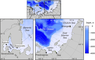Figure 1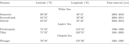Table 1

This study has used long-term hourly sea-level records from 6 stations in the White, Laptev, and Chukchi Seas (Figure 1). The tide gauge stations in the White Sea are Sosnovets, Solovki, and Severodvinsk. Sosnovets is located in Gorlo (the White Sea Throat), Solovki and Severodvinsk stations are located in the entrance to Onega Bay and in the apex of Dvina Bay, respectively. The sea-level records of the White Sea stations cover time interval from 2004 to 2014 (Table 1).

In the Arctic eastern sector, the seasonal variability of harmonic constants was considered at three stations: Tiksi and Preobrajenie in the Laptev Sea and Wrangel in the Chukchi Sea. These data were obtained from the ESIMO portal (Unified State System of Information on the Environment in the World Ocean). The maximum length of the records, from 1977 to 2009, was found at the Tiksi station. Among the series of observations used in the study, there were gaps in the sea level data of various lengths and erroneous values that were eliminated during the initial data processing. The series with high data quality were selected to analyze the tidal characteristics. In the case with the Wrangel station, original series of sea level record from 1977 to 2003 were used to form continues series from 1981 to 1995. And for the Preobrajenie station located in the Khatanga Bay of the Laptev Sea only three-year series (1986–1988) were selected for the analysis (Table 1).

For the analysis of the seasonal variability of tidal harmonics, we performed harmonic analysis of tides [Kondrin, 2008; Pawlowicz et al., 2002] by the least squares method. Altogether, amplitudes and phases for 68 tidal constituents were calculated.

The calculation of the mean tidal annual amplitudes and phases, as well as their standard deviations, was performed using vector averaging of the yearly-computed values [Crawford, 1982; Medvedev et al., 2013]. As a result, the average long-term values of the amplitudes and Greenwich phases were evaluated for individual harmonics at all six stations. To analyze the seasonal variability of tidal harmonics, the average amplitudes and phase lags were calculated for each individual month.

### 3. Spectrum Analysis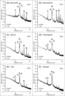Figure 2

Spectral analysis of the sea level oscillations allows to determine the wave energy distribution over the frequencies and to estimate the frequency-selective properties of the water area. Also, the use of the spectral analysis allows to split diurnal and semidiurnal peaks into individual tidal components and investigate the fine structure of tidal peaks [Medvedev et al., 2013]. The spectra were calculated using the fast Fourier transform (FFT) by the Welch's method [Welch, 1967], using the Kaiser-Bessel spectral window with half overlap. The length of the spectral window (segment) $N$ for the three stations in the White Sea was 4096 h (with a spectral resolution $\Delta f = 0.00586$ cpd), for longer series in Tiksi and Wrangel (Figure 2e–f) $N = 8192$ h ($\Delta f = 0.00293$ cpd), and for the Preobrajenie $N = 2048$ h ($\Delta f = 0.0117$ cpd). The number of degrees of freedom $\nu$ depends on the length of the sea level record and on the length of the segment $N$ and $\nu$ varied from 24 (for Preobrajenie) to 52 (for Tiksi).

In Figure 2 the sea level spectra are shown in the frequency range from 0.2 to 12 cpd for all six stations. The spectral energy decreases from low toward high frequencies. Depending on the nature of sea level oscillations, the spectrum can have a continuous nature of the energy distribution (continuum), which is typical for processes with turbulent noise character so as the form of sharp delta-like peaks (discrete spectrum), which corresponds to regular harmonic components with fixed frequencies. The sea level oscillations, induced by variable atmospheric pressure and wind fields at the sea surface are basically random in nature and have a noise spectrum as a continuous function of the frequency.

Tides are clearly pronounced in the sea level spectra in the form of narrow and sharp peaks corresponding to the frequencies of the major tidal harmonics: diurnal K$_1$ (period of 23.93 h), O$_1$ (25.82 h), and semidiurnal M$_2$ (12.42 h), S$_2$ (12.00 h). The semidiurnal tidal peaks noticeably dominate over the diurnal ones in the spectra at all tide gauges of both regions: in the White Sea (Figure 2a–c) and in the seas of the Siberian continental shelf (Figure 2d–f). The White Sea is also characterized by pronounced high-frequency spectrum peaks corresponding to shallow-water tidal constituents (M$_4$, M$_6$, etc.). Shallow-water harmonics are most pronounced in the sea level spectra at the Severodvinsk, located in the head of the Dvina Bay. The diurnal constituent O$_1$ is well pronounced at the tide gauges in the Laptev and Chukchi seas, and is practically absent in the White Sea.

An interesting feature was observed within the semidiurnal frequency band (Figure 2). In addition to the sharp discrete tidal peaks corresponding to the harmonics M$_2$, S$_2$, N$_2$, in Figure 2 an increase of the continuous part of the spectrum (continuum) at the frequencies from 1.6 to 2.4 cpd was also revealed. This feature was at first described in [Munk et al., 1965] and was called "tidal cusps". The "tidal cusps" probably appeared in the sea level spectrum in the Arctic because of seasonal variability of the amplitudes and phases of the major semidiurnal harmonics.

### 4. Harmonic Analysis of Tides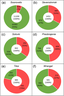Figure 3

The contribution of tides to the total sea level variations was assessed before tides were analyzed. Figure 3 shows the variance relations, characterizing the sea level energy of tidal and residual (non-tidal) oscillations for all stations.

The energy of total sea level oscillations reaches its maximum at Sosnovets in the White Sea strait Gorlo (11,209 cm$^2$), where the variance of tides reaches 10,383 cm$^2$, which is an order of magnitude greater than at the other stations (Figure 3). In general, in the White Sea, the tidal energy everywhere strongly prevails over non-tidal energy and is significantly larger than at the Siberian continental shelf stations.

At some of the islands, such as Solovki in the White Sea (Figure 3c), and Wrangel on the Siberian continental shelf (Figure 2f), the total sea level variance is noticeably smaller: 842 cm$^2$ and 581 cm$^2$, respectively. In contrast, the absolute tidal energy at Preobrajenie island is higher than at Solovki, and the total energy of all sea level oscillations is comparable to that at Severodvinsk (1452 cm$^2$), reaching 1450 cm$^2$. This is due to the position of the island at the entrance of narrow Khatanga Bay, where the tidal energy accumulates.

The absolute energy of residual oscillations at Tiksi (783 cm$^2$) is the second only to Sosnovets (826 cm$^2$). In Tiksi Bay, in general, there is a substantial predominance of residual oscillations (79%) over tidal, with the latter making the minimum contribution to the variance among all stations. This is due to the fact that the maximum surge range among all stations is observed in Tiksi Bay and near the delta of the Lena River, where seasonal sea level oscillations make a significant contribution.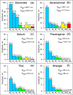Figure 4

In Figure 4, the amplitudes of the major tidal constituents at six tide gauges are shown. The principal lunar semidiurnal constituent M$_2$ dominates at all stations. Also, at all stations pronounced amplitudes have semidiurnal constituents S$_2$ and N$_2$. The amplitudes of the main diurnal harmonics K$_1$ and O$_1$ are much smaller than the amplitudes of major semidiurnal harmonics.

The harmonic constituents can be used to describe common non-harmonic parameters of tides: form factor $(F)$, the mean spring range ($R_{\mathrm{spr}}$), the maximum predicted tidal range $(R_{\mathrm{abs}}$) and the character of the shallow-water tides expressed by the ratio of M$_4$/M$_2$ waves.

The relative importance of diurnal and semidiurnal tidal constituents is commonly expressed in terms of the form factor [Pugh and Woodworth, 2014]:

\begin{eqnarray*} F= \frac{H_{\mathrm{K}_1} +H_{\mathrm{O}_1}}{H_{\mathrm{M}_2}+ H_{\mathrm{S}_2}} \end{eqnarray*}

At all stations in the White Sea, as well as at the stations of Wrangel and Preobrajenie, $F \leq 0.25$ and the tide is semidiurnal. At Tiksi Bay, $F$ is close to 0.3, which corresponds to the mixed tidal type, with prevailing semidiurnal constituents.

The mean value of the doubled sum of the major semidiurnal tidal amplitudes

\begin{eqnarray*} R_{\mathrm{spr}} = 2 (H_{\mathrm{M}_2} + H_{\mathrm{S}_2}) \end{eqnarray*}

makes it possible to estimate the magnitude of the semidiurnal spring tide. Among the stations located on the Siberian continental shelf, the highest value of the mean spring range is observed at Preobrajenie, where $R_{\mathrm{spr}} \sim 96$ cm.

The maximum $R_{\mathrm{abs}}$ was calculated as the maximum difference between high and low water during one diurnal lunar cycle according to the predicted 18.6-year tidal record. The values of parameter $R_{\mathrm{abs}}$ are much higher than the mean spring range $R_{\mathrm{spr}}$: at the station Sosnovets $R_{\mathrm{abs}}$ reaches 429 cm, which is 68 cm larger than $R_{\mathrm{spr}}$, and at the stations Severodvinsk $(R_{\mathrm{abs}} = 172$ cm, $R_{\mathrm{spr}} = 101$ cm) and Tiksi $(R_{\mathrm{abs}} = 49$ cm, $R_{\mathrm{spr}} = 31$ cm), $R_{\mathrm{abs}}$ values are more than 50% higher than $R_{\mathrm{spr}}$. This increase in the maximum possible tidal magnitude is associated with both seasonal modulation of tides, which is more typical for the stations of the eastern sector of the Arctic, and with the influence of secondary tidal waves, such as shallow-water harmonics for stations in the White Sea.

The White Sea has complex boundaries and bottom relief. With the nonlinear transformation of the main semidiurnal waves, the high-frequency tidal harmonics M$_4$, MS$_4$, and M$_6$ become more significant. The largest values of these constituents are achieved in shallow bays. Thus, at Severodvinsk, the M$_4$ and M$_6$ amplitudes can be compared with the amplitude of S$_2$, reaching $\sim 9$ cm. Due to the presence of shallow-water harmonics in the White Sea, the rise and fall of the semidiurnal tide becomes asymmetrical. As a result, they delay the onset of high water and in some places even leads to the appearance of high-water false peaks (the phenomenon called "manikha"). The M$_4$/M$_2$ ratio enables us to evaluate the asymmetry of the tidal rise and fall durations. According to [Gidrometeoizdat, 1991], at M$_4$/M$_2$ = 0.04 this difference will be 30 minutes, and for M$_4$/M$_2 = 0.08$ about 1 hour. For Sosnovets, the ratio M$_4$/M$_2 = 0.06$, and for Solovki and Severodvinsk stations M$_4$/M$_2 = 0.11$ and 0.21, respectively, indicating the significant deformation of the semidiurnal tidal level at these stations. At the Siberian continental shelf stations, the M$_4$/M$_2$ values do not exceed 0.02 (Tiksi).

### 5. Seasonal Variability of the Main Tidal Waves

Mean values of the amplitude and phase of the main tidal harmonics were calculated together with their standard deviations. These values were obtained for each month of the multi-year series. The data from the Preobrajenie station were not used in this analysis, due to a relatively short series (3 years).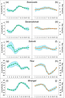Figure 5

The seasonal variations of amplitudes and phases of the major semidiurnal constituent M$_2$ for the five stations are shown in Figure 5.

The stations on the Siberian continental shelf have significantly stronger seasonal variations of the M$_2$ amplitudes and phases than the stations in the White Sea (Figure 5). In the Siberian continental shelf areas, the seasonal deviations of the tidal constants from the mean annual values reach 42% (for amplitude, Figure 5i) and up to 49% (for phase, Figure 5h), while the maximum deviations from the mean amplitudes and phases for the White Sea stations are only 5% (Figure 5c) and 2% (Figure 5f), respectively.

The seasonal modulation for the M$_2$ is expressed as the difference between the maximum and minimum mean monthly values (% of their deviations from the mean annual value) reaches the highest 63% at Wrangel and 43% at Tiksi. At stations in the White Sea, the maximum of 9% modulation was obtained for Severodvinsk. For the Sosnovets and Solovki stations, it is approximately 7%.

Nevertheless, seasonal variations at Sosnovets, Severodvinsk (Figure 5a–d), and Wrangel (Figure 5i–j) have a similar seasonal variability in amplitude with the maximum in July–August, while the minimum corresponds to the period in March–April when the ice cover was at maximum. This confirms the conclusions of Proshutinsky  on the effect of the ice cover on the tide. At Wrangel Island, the tidal phase increases (Figure 5j) during the period of maximum ice development (April).

At Solovki and Tiksi (Figure 5e–h), the so-called "anomalous seasonal variability" of the M$_2$ tidal constituent is observed. It means that the amplitude increases in spring (March–May) and decreases in summer (May–August). Also, the minimum amplitudes in December take place at Tiksi (Figure 5g). This anomalous seasonal variation is apparently associated with the location of amphidromic systems in the Laptev Sea during the year and with the local tidal features at the Solovetsky Islands.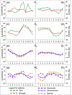Figure 6

In addition to the principal lunar harmonic M$_2$, the study considers seasonal variations of other tidal components: (Figure 6): semidiurnal S$_2$ for all stations except Preobrajenie, diurnal K$_1$ for the Siberian continental shelf stations and shallow-water M$_4$ for stations in the White Sea.

The seasonal modulation of the principal solar tidal harmonic S$_2$ for the Siberian continental shelf stations reaches 92% (Tiksi) and 87% (Wrangel), while for the stations from the White Sea, this modulation is in the range of 12–19% (Figure 6e–f). Maximum amplitudes of S$_2$ at Tiksi and Wrangel occur in August, while the minima occur in April–May at Wrangel and in December at Tiksi, coinciding with the minima of the M$_2$ amplitudes. The seasonal variation of S$_2$ phases, in general, is similar at these stations (Figure 6d).

In the White Sea, seasonal changes of the S$_2$ harmonic constituent proceed concurrently at all three stations. The amplitude maxima are observed in December and January, and their minima are in the summer months (May–July). As is known, the harmonic S$_2$ is substantially influenced by solar radiation [Pugh and Woodworth, 2014], which obviously contributes to its annual modulation.

At Wrangel Island, the lunar-solar declination tidal constituent K$_1$ (Figure 6a–b) has less pronounced seasonal variation than semidiurnal tides, with the seasonal amplitude modulation of this constituent $\sim 44$% (in comparison with 63 and 87% for the M$_2$ and S$_2$ modulation, respectively). The maximum variability of this harmonic, up to 114%, is observed at Tiksi. It is also interesting to note that the extrema for the seasonal variation of the K$_1$ amplitude (Figure 6a) are almost the opposite to that one of M$_2$ (Figure 5g, Figure 5i), with the seasonal amplitude variation being in antiphase both for K$_1$ and M$_2$ at stations Tiksi and Wrangel.

For the first time, the seasonal variability of shallow-water harmonics M$_4$ and M$_6$ in the White and Barents Seas was considered by Voinov . The results of our study suggest that the amplitudes of these harmonics on the Siberian continental shelf seas are small, so are the seasonal variations of their amplitudes and phases. Therefore we consider these variations only for the White Sea stations (Figure 6g–h). The amplitude modulation (Figure 6g) for stations in the White Sea ranges from 11% (Solovki) to 13% (Sosnovets). The amplitude maxima of the harmonic M$_4$ in the White Sea occur in the summer months, while at Solovki there is an additional peak in January. The M$_4$ amplitude minima at Sosnovets and Severodvinsk take place in November–December, and at Solovki in March. The intra-annual phase changes vary from 11° (Sosnovets, Severodvinsk) to 21° (Solovki).

### 6. Seasonal Variability of the Nonharmonic Tidal Characteristics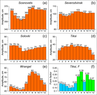Figure 7

The seasonal variations of a mean spring range $R_{\mathrm{spr}}$ are presented in Figure 7. In general, their extrema correspond to the extrema of the seasonal M$_2$ variations with an additional maxima in December–January at the White Sea stations, associated with the maxima in the S$_2$ seasonal variations. The most notable seasonal changes of $R_{\mathrm{spr}}$ are observed at Wrangel, where $R_{\mathrm{spr}}$ varies from a minimum of 42 cm in April to a maximum of 82 cm in August. Such large relative range (more than 70%) of seasonal changes is due to the in-phase seasonal variations of the M$_2$ (Figure 5i) and S$_2$ (Figure 6c) amplitudes registered at this station. At Tiksi, where the seasonal variation of the harmonics M$_2$ (Figure 5g) and S$_2$ (Figure 6c) is in antiphase, the annual changes in $R_{\mathrm{spr}}$ do not exceed 40% despite high seasonal variability of each of these harmonics. At stations in the White Sea, the relative changes in the seasonal variation of $R_{\mathrm{spr}}$ are in the range of 4–11%.

The seasonal changes of the tide form factor $(F)$ at Tiksi, where significant seasonal variability of both semidiurnal and pronounced diurnal tides takes place, are shown in Figure 7f.

Semidiurnal tides $(F \leq 0.25)$ are observed from January to June and in September, when the harmonics S$_2$ and K$_1$ in their seasonal variation are in antiphase with the principal lunar harmonic M$_2$. When the M$_2$ has a smaller amplitude, the ratio $F$ exceeds 0.25 (July–August, October–December), reaching 0.39 in July, which indicates the "mixed semidiurnal tidal regime" at this time.

### 7. Discussion

The results of the present study revealed specific tidal features in the Arctic Ocean and confirmed the hypothesis of Wiese , Proshutinsky , St-Laurent et al.  and Müller et al.  about the significant influence of the ice-cover on tides in the Arctic seas. This effect was found dominant for a number of stations (Severodvinsk, Sosnovets and Wrangel) as indicated by the seasonal variation of the major tidal harmonic M$_2$. However, for some other stations (Solovki and Tiksi), the seasonal variability of tides cannot be explained only by the effect of the ice cover. Location of these stations near amphidromic systems [Gidrometeoizdat, 1991; Kowalik and Proshutinsky, 1993] is the most likely cause of their anomalous (not related to the ice cover) seasonal variability.

Seasonal S$_2$ variations are similar at the Siberian continental shelf stations and almost identical at the White Sea stations. This indicates the prevailing influence of hydrometeorological conditions on the tidal harmonic. The ice cover effect is more pronounced at the Siberian continental shelf, while in the White Sea the S$_2$ modulation appears to be caused by the radiational effects with increasing role of the breeze circulation in summer.

The seasonal variations of the diurnal harmonic K$_1$ also mainly depend on the hydrometeorological conditions, with the maximum relative changes at Tiksi (up to 114%). Specifically, these strong seasonal changes in the K$_1$ amplitude are responsible for the seasonal changes in the tidal type at this station (from "semidiurnal" to "mixed semidiurnal"). The seasonal variations of the shallow-water constituent M$_4$ are similar to those of the principal lunar component M$_2$ with the amplitude maxima in the summer months, although with specific peculiarities at each station.

The nature of the seasonal variations of the mean spring range $R_{\mathrm{spr}}$ depends primarily on how consistent are the seasonal variations of the harmonics M$_2$ and S$_2$. Seasonal variability of $R_{\mathrm{spr}}$ at Wrangel and Tiksi stations is a good example of how synchronous (or asynchronous) seasonal variations of the major semidiurnal harmonics can affect the total magnitude of the semidiurnal spring tide. The maximum tide, $R_{\mathrm{abs}}$, characterized by the largest difference between the low and high water within the diurnal tidal cycle over the nodal period (18.6 years), is practically equal to the maximum observed tidal range, i.e. to the difference between the minimum and maximum tidal levels for the entire period. The $R_{\mathrm{abs}}$ value is larger than the mean spring tide by 20–70% for various stations.

To assess the contribution of the seasonal variations of the main harmonics, the variance of tidal and residual sea-level oscillations was calculated. The seasonal variability was accounted by its interpretation using annual and semiannual harmonic satellites – group of constituents which frequencies are close to the major tidal constituents [Voinov, 2003]. For each of the major tidal waves we chosed groups of such satellites that modulate it with an annual and semiannual periodicity. The variance of tidal and residual sea-level oscillations was calculated taking into account the seasonal variation of the main harmonics and without it.

The annual sea level observation series, which are used in calculations, allow to resolve the characteristics of these secondary waves, to compare their contribution to the tidal variance, and to estimate the changes in the residual variance for the stations under investigation. For the Sosnovets (the White Sea) station, where the tide has a maximum value, the variance of tidal sea level oscillations increases by 1.2%, and the residual variance decreases by almost 40%. For the Siberian continental shelf stations, Tiksi and Wrangel, the presence of satellite waves in the calculations increases tidal variance by 26% and 9%, respectively, while the non-tidal variance decreases by 10% for Tiksi and 21% for Wrangel.

### 8. Conclusions

Mean annual harmonic and nonharmonic characteristics of tides were obtained at six stations in the Arctic seas of Russia (White, Laptev, and Chukchi). The spatial distribution of amplitudes of the major tidal harmonics was examined and shown. The shallow-water high-frequency harmonics contribute significantly to the tidal oscillations in the White Sea, where the tidal amplitudes are almost an order of magnitude higher than in the seas of the Siberian continental shelf. In contrast, in the Siberian seas, the diurnal tides are relatively more important. At Tiksi the main diurnal harmonics are approximately a quarter of the main semidiurnal harmonics, which results in changes of the tide form factor (from "semidiurnal" to "mixed semidiurnal"). The mean value of the spring tide ($R_{\mathrm{spr}}$), was found ranging from 31 cm (Tiksi) to 361 cm (Sosnovets), while the maximum possible tide, $R_{\mathrm{abs}}$ ranges from 49 to 428 cm. The latter tidal characteristics are quite important for practical needs.

Significant differences in the seasonal variability of harmonic constituents, both between individual stations and between the two examined water regions (the White Sea and the Siberian continental shelf) are evident. The seasonal modulation of the M$_2$ is only 7–9% at the White Sea stations, while at Wrangel and Tiksi it reaches 63 and 43%, respectively. At the same time, there is a similarity of seasonal changes at two White Sea stations (Sosnovets and Severodvinsk) and at Wrangel in the Chukchi Sea, which appears to be associated with the dependence of the M$_2$ tidal magnitude on the development of ice cover. What is more, there is a similarity between Solovki and Tiksi, where the M$_2$ seasonal variations are caused by the local features and seasonal displacements of amphidromic systems.

Estimation of seasonal variability of other important tidal harmonics (K$_1$, S$_2$, and M$_4$) also revealed the noteworthy features. The seasonal variations of the S$_2$ amplitudes are synchronic for all White Sea stations and also all Siberian continental shelf stations. In contrast, the seasonal changes of the K$_1$ amplitude are in antiphase at Tiksi and Wrangel. Besides, the extremes for this diurnal harmonic and for the semidiurnal harmonic M$_2$ are opposite in time. Estimation of seasonal variability of the shallow-water harmonic M$_4$ in the White Sea showed good agreement with M$_2$. At the same time, there is an increase of seasonal changes in shallow-water tides (up to 13%), as compared to the diurnal tides. This statement is also true for the harmonics K$_1$ and S$_2$, the range of which can reach 114% and 92%, respectively. However, these estimates are associated with the large scatter of standard deviation of the seasonal range magnitude, which makes the seasonal variations of these harmonics unstable. The results of the present study have shown that the prediction of tidal sea level oscillations requires taking into account the seasonal variability of the tidal harmonics.

#### Acknowledgments

The results of Section 3 were obtained in the framework of the State assignment of FASO Russia (Theme No. 0149-2018-0015). The studies presented Section 4 were supported by the RSF grant (Project No. 14-50-00095), the results of Section 5 by the RFBR grants (Project No. 17-05-41144 and 18-05-60250) and in Section 6 by the RSF grant (Project No. 14-37-00038).

### References

Cartwright, D. E. (1968), A unified analysis of tides and surges round north and east Britain, Phil. Trans. Royal Soc., 263, no. 1134, p. 1–55, https://doi.org/10.1098/rsta.1968.0005.

Corkan, R. H. (1934), An annual perturbation in the range of tide, Proc. R. Soc. Lond., Ser. A., 144, no. 853, p. 537–559.

Crawford, W. R. (1982), Analysis of fortnightly and monthly tides, International Hydrographic Review, 59, no. 1, p. 131–141.

Defant, A. (1961), Physical Oceanography, Vol. 2, 598 pp., Pergamon Press, Oxford.

Dvorkin, E. N. (1970), Tides, Soviet Arctic, p. 191–197, Nauka, Moscow.

Foreman, M. G. G., R. A. Walters, R. F. Henry, C. P. Keller, A. G. Dolling (1995), A tidal model of eastern Juan de Fuca Strait and the southern Strait of Georgia, J. Geophys., 100, p. 721–740, https://doi.org/10.1029/94JC02721.

Gidrometeoizdat, (1991), Hydrometeorology and Hydrochemistry of Seas of the Soviet Union, Vol. 2, The White Sea, No. 1, 240 pp., Gidrometeoizdat, Leningrad.

Huess, V., O. B. Andersen (2001), Seasonal variation in the main tidal constituent from altimetry, Geophys. Res. Lett., 28, p. 567–570, https://doi.org/10.1029/2000GL011921.

Kagan, B. A., E. V. Sofina (2010), Ice-induced seasonal variability of tidal constants in the Arctic Ocean, Continental Shelf Research, 30, no. 6, p. 643–647, https://doi.org/10.1016/j.csr.2009.05.010.

Kondrin, A. T. (2008), Methods of harmonic analysis of tides, Vestnik MGU. Ser. 5. Geogr., 5, p. 26–30.

Kowalik, Z., A. Yu. Proshutinsky (1993), The diurnal tides in the Arctic Ocean, J. Geophys. Res., 98, p. 16,449–16,468, https://doi.org/10.1029/93JC01363.

Medvedev, I. P., A. B. Rabinovich, E. A. Kulikov (2013), Tidal oscillations in the Baltic Sea, Oceanology, 53, no. 5, p. 526–538, https://doi.org/10.1134/S0001437013050123.

Müller, M., J. Y. Cherniawsky, M. G. G. Foreman, J. S. Storch (2014), Seasonal variation of the M$_2$ tide, Ocean Dynamics, 64, no. 2, p. 159–177, https://doi.org/10.1007/s10236-013-0679-0.

Munk, W. H., B. Zetler, G. W. Groves (1965), Tidal cusps, Geophysical Journal of the Royal Astronomical Society, 10, no. 2, p. 211–219, https://doi.org/10.1111/j.1365-246X.1965.tb03062.x.

Pawlowicz, R., B. Beardsley, S. Lentz (2002), Classical tidal harmonic analysis including error estimates in MATLAB using T$_$TIDE, Computers &amp; Geosciences, 28, no. 8, p. 929–937.

Proshutinsky, A. Yu. (1993), The Arctic Ocean level oscillations, 216 pp., Gidrometeoizdat, St-Petersburg.

Pugh, D. T., P. L. Woodworth (2014), Sea-level Science: Understanding Tides, Surges, Tsunamis and Mean Sea-level Changes, 396 pp., Cambridge University Press, Cambridge.

Shevchenko, G. V. (1996), Quasi-periodic seasonal variability of tide harmonic constants in the Northwestern Sea of Okhotsk, Russian Meteorology and Hydrology, 8, p. 50–57.

St-Laurent, P., F. J. Saucier, J. F. Dumais (2008), On the modification of tides in a seasonally ice-covered sea, J. Geophys. Res., 113, p. C11014, https://doi.org/10.1029/2007JC004614.

Voinov, G. N. (2003), New interpretation of seasonal variability of tides of the Russian Arctic Seas, Russian Meteorology and Hydrology, 9, p. 42–51.

Voinov, G. N. (2007), Seasonal variability of the harmonic constants of the quarter-diurnal and sixth-diurnal constituents in the Barents Sea and White Sea, Russian Meteorology and Hydrology, 4, p. 252–261, https://doi.org/10.3103/S106837390704005X.

Voinov, G. N. (2016), Tides in the Gulf of Ob (Kara Sea). II. The influence of ice cover on the characteristics of the tides, Proceedings of the RSHU, 44, p. 43–63.

Welch, P. D. (1967), The use of fast Fourier transform for the estimation of power spectra: a method based on time averaging over short, modified periodograms, IEEE Trans. Audio Electroacoust., 15, no. 2, p. 70–73, https://doi.org/10.1109/TAU.1967.1161901.

Wiese, W. J. (1936), Preface to the "Materials for the study of the tides of the Arctic seas of the USSR. Part 2", Transactions of the Arctic Institute, 52, p. 5–7.

Received 8 June 2018; accepted 24 June 2018; published 18 October 2018.Citation: Kulikov M. E., I. P. Medvedev, A. T. Kondrin (2018), Seasonal variability of tides in the Arctic Seas, Russ. J. Earth Sci., 18, ES5003, doi:10.2205/2018ES000633.

Generated from LaTeX source by ELXpaper, v.1.5 software package.